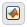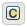## Modify the Action Language for a Chart

Stateflow® charts in Simulink® models have an action language property that defines the syntax for state and transition actions. An icon in the lower-left corner of the chart canvas indicates the action language for the chart.

•MATLAB® as the action language.

•C as the action language.

You can change the action language of a chart in the Action Language box of the Chart properties dialog box. For more information, see Differences Between MATLAB and C as Action Language Syntax.

### Change the Default Action Language

MATLAB is the default action language syntax for new Stateflow charts. To create a chart that uses C as the action language, enter:

`sfnew -C`

To change the default action language of new charts, use the `sfpref` function. For example, to set C as the action language for new charts, enter:

`sfpref(ActionLanguage="C");`

### Auto Correction When Using MATLAB as the Action Language

Stateflow charts that use MATLAB as the action language automatically correct common C constructs to MATLAB syntax:

• Increment and decrement operations such as `a++` and `a--`. For example, `a++` is changed to `a = a+1`.

• Assignment operations such as `a += b`, ```a –= b```, `a *= b`, and ```a /= b```. For example, `a += b` is changed to `a = a+b`.

• Evaluation operations such as `a != b` and `!a`. For example, `a != b` is changed to `a ~= b`.

• Comment markers `//` and `/* */` are changed to `%`.

To disable this preference, use the `sfpref` function:

`sfpref(EnableLabelAutoCorrectionForMAL=false);`

### C to MATLAB Syntax Conversion

For nonempty charts, after you change the action language property from C to MATLAB, a notification appears at the top of the chart. The notification provides the option to convert some of the C syntax to MATLAB syntax. In the notification, click the link to have Stateflow convert syntax in the chart. C syntax constructs that are converted include:

• Zero-based indexing.

• Increment and decrement operations such as `a++` and `a--`. For example, `a++` is changed to `a = a+1`.

• Assignment operations such as `a += b`, ```a –= b```, `a *= b`, and `a /= b`. For example, `a += b` is changed to ```a = a+b```.

• Binary operations such as `a %% b`, ```a >> b```, and `a << b`. For example, `a %% b` is changed to `rem(a,b)`.

• Bitwise operations such as `a ^ b`, ```a & b```, and `a | b`. For example, if the chart property Enable C-bit operations is selected, then `a ^ b` is changed to `bitxor(a,b)`.

• C style comment markers. For example, `//` and `/* */` are changed to `%`.

If the chart contains C constructs that cannot be converted to MATLAB, Stateflow shows a message in a dialog box. Click on the warnings link to display the warnings in the Diagnostic Viewer. Choose whether or not to continue with the conversion of supported syntax. C constructs not converted to MATLAB include:

• Explicit type casts with `cast` and `type`

• Operators such as `&`, `*` and `:=`

• Custom data

• Access to workspace variables using `ml` operator

• Functions not supported in code generation

• Hexadecimal and single precision notation

• Context-sensitive constants